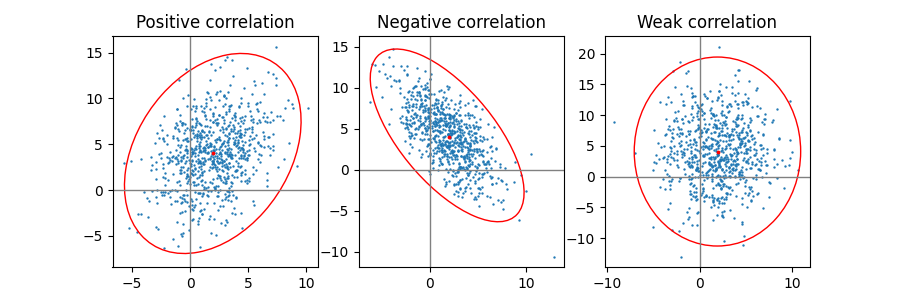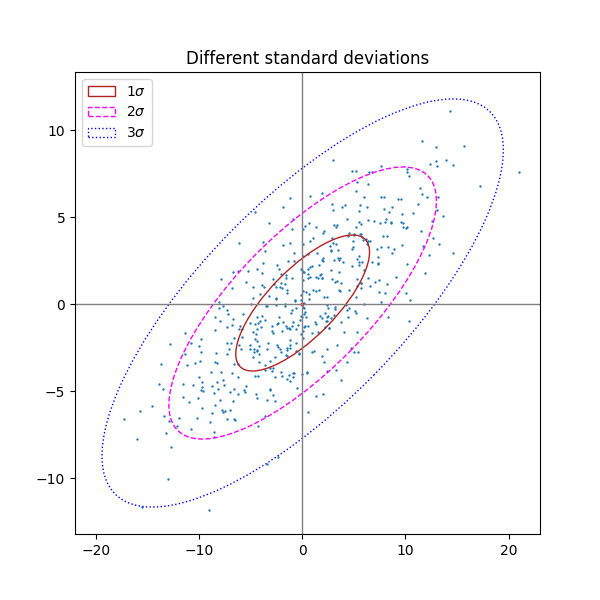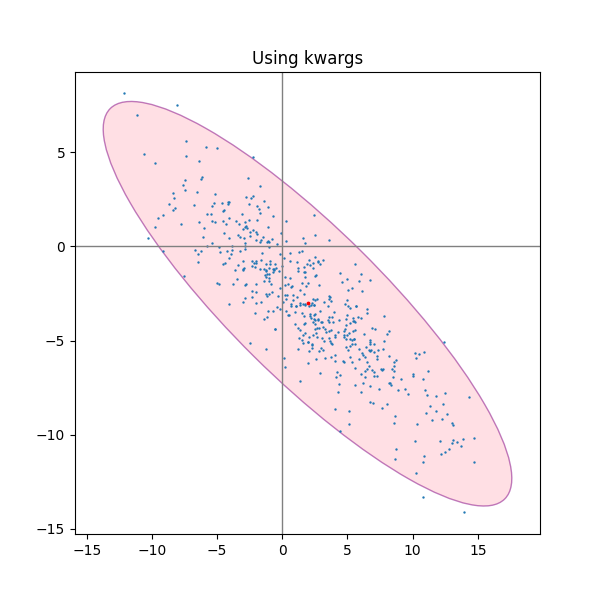# Plot a confidence ellipse of a two-dimensional dataset¶

This example shows how to plot a confidence ellipse of a two-dimensional dataset, using its pearson correlation coefficient.

The approach that is used to obtain the correct geometry is explained and proved here:

https://carstenschelp.github.io/2018/09/14/Plot_Confidence_Ellipse_001.html

The method avoids the use of an iterative eigen decomposition algorithm and makes use of the fact that a normalized covariance matrix (composed of pearson correlation coefficients and ones) is particularly easy to handle.

import numpy as np
import matplotlib.pyplot as plt
from matplotlib.patches import Ellipse
import matplotlib.transforms as transforms


## The plotting function itself¶

This function plots the confidence ellipse of the covariance of the given array-like variables x and y. The ellipse is plotted into the given axes-object ax.

The radiuses of the ellipse can be controlled by n_std which is the number of standard deviations. The default value is 3 which makes the ellipse enclose 99.7% of the points (given the data is normally distributed like in these examples).

def confidence_ellipse(x, y, ax, n_std=3.0, facecolor='none', **kwargs):
"""
Create a plot of the covariance confidence ellipse of *x* and *y*.

Parameters
----------
x, y : array-like, shape (n, )
Input data.

ax : matplotlib.axes.Axes
The axes object to draw the ellipse into.

n_std : float
The number of standard deviations to determine the ellipse's radiuses.

**kwargs
Forwarded to ~matplotlib.patches.Ellipse

Returns
-------
matplotlib.patches.Ellipse
"""
if x.size != y.size:
raise ValueError("x and y must be the same size")

cov = np.cov(x, y)
pearson = cov[0, 1]/np.sqrt(cov[0, 0] * cov[1, 1])
# Using a special case to obtain the eigenvalues of this
# two-dimensionl dataset.
facecolor=facecolor, **kwargs)

# Calculating the stdandard deviation of x from
# the squareroot of the variance and multiplying
# with the given number of standard deviations.
scale_x = np.sqrt(cov[0, 0]) * n_std
mean_x = np.mean(x)

# calculating the stdandard deviation of y ...
scale_y = np.sqrt(cov[1, 1]) * n_std
mean_y = np.mean(y)

transf = transforms.Affine2D() \
.rotate_deg(45) \
.scale(scale_x, scale_y) \
.translate(mean_x, mean_y)

ellipse.set_transform(transf + ax.transData)


## A helper function to create a correlated dataset¶

Creates a random two-dimesional dataset with the specified two-dimensional mean (mu) and dimensions (scale). The correlation can be controlled by the param 'dependency', a 2x2 matrix.

def get_correlated_dataset(n, dependency, mu, scale):
latent = np.random.randn(n, 2)
dependent = latent.dot(dependency)
scaled = dependent * scale
scaled_with_offset = scaled + mu
# return x and y of the new, correlated dataset
return scaled_with_offset[:, 0], scaled_with_offset[:, 1]


## Positive, negative and weak correlation¶

Note that the shape for the weak correlation (right) is an ellipse, not a circle because x and y are differently scaled. However, the fact that x and y are uncorrelated is shown by the axes of the ellipse being aligned with the x- and y-axis of the coordinate system.

np.random.seed(0)

PARAMETERS = {
'Positive correlation': [[0.85, 0.35],
[0.15, -0.65]],
'Negative correlation': [[0.9, -0.4],
[0.1, -0.6]],
'Weak correlation': [[1, 0],
[0, 1]],
}

mu = 2, 4
scale = 3, 5

fig, axs = plt.subplots(1, 3, figsize=(9, 3))
for ax, (title, dependency) in zip(axs, PARAMETERS.items()):
x, y = get_correlated_dataset(800, dependency, mu, scale)
ax.scatter(x, y, s=0.5)

ax.axvline(c='grey', lw=1)
ax.axhline(c='grey', lw=1)

confidence_ellipse(x, y, ax, edgecolor='red')

ax.scatter(mu, mu, c='red', s=3)
ax.set_title(title)

plt.show()## Different number of standard deviations¶

A plot with n_std = 3 (blue), 2 (purple) and 1 (red)

fig, ax_nstd = plt.subplots(figsize=(6, 6))

dependency_nstd = [[0.8, 0.75],
[-0.2, 0.35]]
mu = 0, 0
scale = 8, 5

ax_nstd.axvline(c='grey', lw=1)
ax_nstd.axhline(c='grey', lw=1)

x, y = get_correlated_dataset(500, dependency_nstd, mu, scale)
ax_nstd.scatter(x, y, s=0.5)

confidence_ellipse(x, y, ax_nstd, n_std=1,
label=r'$1\sigma$', edgecolor='firebrick')
confidence_ellipse(x, y, ax_nstd, n_std=2,
label=r'$2\sigma$', edgecolor='fuchsia', linestyle='--')
confidence_ellipse(x, y, ax_nstd, n_std=3,
label=r'$3\sigma$', edgecolor='blue', linestyle=':')

ax_nstd.scatter(mu, mu, c='red', s=3)
ax_nstd.set_title('Different standard deviations')
ax_nstd.legend()
plt.show()## Using the keyword arguments¶

Use the kwargs specified for matplotlib.patches.Patch in order to have the ellipse rendered in different ways.

fig, ax_kwargs = plt.subplots(figsize=(6, 6))
dependency_kwargs = [[-0.8, 0.5],
[-0.2, 0.5]]
mu = 2, -3
scale = 6, 5

ax_kwargs.axvline(c='grey', lw=1)
ax_kwargs.axhline(c='grey', lw=1)

x, y = get_correlated_dataset(500, dependency_kwargs, mu, scale)
# Plot the ellipse with zorder=0 in order to demonstrate
# its transparency (caused by the use of alpha).
confidence_ellipse(x, y, ax_kwargs,
alpha=0.5, facecolor='pink', edgecolor='purple', zorder=0)

ax_kwargs.scatter(x, y, s=0.5)
ax_kwargs.scatter(mu, mu, c='red', s=3)
ax_kwargs.set_title('Using kwargs')